Courses

# Flow Rate and Its Relation to Velocity Physics Notes | EduRev

## Physics : Flow Rate and Its Relation to Velocity Physics Notes | EduRev

The document Flow Rate and Its Relation to Velocity Physics Notes | EduRev is a part of the Physics Course Basic Physics for IIT JAM.
All you need of Physics at this link: Physics

Flow rateQ is defined to be the volume of fluid passing by some location through an area during a period of time, as seen in. In symbols, this can be written as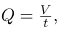where V is the volume and t is the elapsed time.
The SI unit for flow rate is m3/s, but a number of other units for Q are in common use. For example, the heart of a resting adult pumps blood at a rate of 5.00 liters per minute (L/min). Note that a but a number of other units for Q are in common use. For example, the heart of a resting adult pumps blood at a rate of 5.00 liters per minute (L/min). Note that a liter (L) is 1/1000 of a cubic meter or 1000 cubic centimeters (10-3 m3 or 103). In this text we shall use whatever metric units are most convenient for a given situation.
Flow rate is the volume of fluid per unit time flowing past a point through the area A. Here the shaded cylinder of fluid flows past point P in a uniform pipe in time t. The volume of the cylinder is Ad and the average velocity is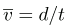so that the flow rate is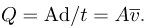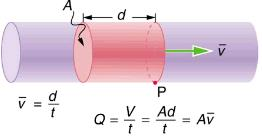Calculating Volume from Flow Rate: The Heart Pumps a Lot of Blood in a Lifetime
How many cubic meters of blood does the heart pump in a 75-year lifetime, assuming the average flow rate is 5.00 L/min?
Strategy
Time and flow rate Q are given, and so the volume V can be calculated from the definition of flow rate.
Solution
Solving Q=V/t for volume gives
V = Qt.
Substituting known values yields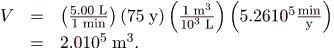Discussion
This amount is about 200,000 tons of blood. For comparison, this value is equivalent to about 200 times the volume of water contained in a 6-lane 50-m lap pool.
Flow rate and velocity are related, but quite different, physical quantities. To make the distinction clear, think about the flow rate of a river. The greater the velocity of the water, the greater the flow rate of the river. But flow rate also depends on the size of the river. A rapid mountain stream carries far less water than the Amazon River in Brazil, for example. The precise relationship between flow rate Q and velocity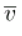is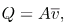where A is the cross-sectional area andis the average velocity. This equation seems logical enough. The relationship tells us that flow rate is directly proportional to both the magnitude of the average velocity (hereafter referred to as the speed) and the size of a river, pipe, or other conduit. The larger the conduit, the greater its cross-sectional area. Illustrates how this relationship is obtained. The shaded cylinder has a volume
which flows past the point P in a time t. Dividing both sides of this relationship by t gives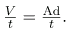We note that Q=V/t and the average speed is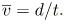Thus the equation becomes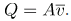Shows an incompressible fluid flowing along a pipe of decreasing radius. Because the fluid is incompressible, the same amount of fluid must flow past any point in the tube in a given time to ensure continuity of flow. In this case, because the cross-sectional area of the pipe decreases, the velocity must necessarily increase. This logic can be extended to say that the flow rate must be the same at all points along the pipe. In particular, for points 1 and 2,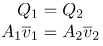This is called the equation of continuity and is valid for any incompressible fluid. The consequences of the equation of continuity can be observed when water flows from a hose into a narrow spray nozzle: it emerges with a large speed—that is the purpose of the nozzle. Conversely, when a river empties into one end of a reservoir, the water slows considerably, perhaps picking up speed again when it leaves the other end of the reservoir. In other words, speed increases when cross-sectional area decreases, and speed decreases when cross-sectional area increases.
When a tube narrows, the same volume occupies a greater length. For the same volume to pass points 1 and 2 in a given time, the speed must be greater at point 2. The process is exactly reversible. If the fluid flows in the opposite direction, its speed will decrease when the tube widens. (Note that the relative volumes of the two cylinders and the corresponding velocity vector arrows are not drawn to scale.)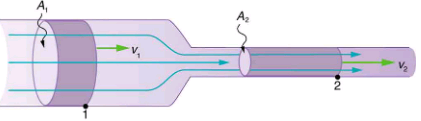Since liquids are essentially incompressible, the equation of continuity is valid for all liquids. However, gases are compressible, and so the equation must be applied with caution to gases if they are subjected to compression or expansion.
Calculating Fluid Speed: Speed Increases When a Tube Narrows
A nozzle with a radius of 0.250 cm is attached to a garden hose with a radius of 0.900 cm. The flow rate through hose and nozzle is 0.500 L/s. Calculate the speed of the water
(a) in the hose and
(b) in the nozzle.
Strategy
We can use the relationship between flow rate and speed to find both velocities. We will use the subscript
1 for the hose and
2 for the nozzle.
Solution for (a)
First, we solve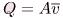for v1 and note that the cross-sectional area is A = πr2, yielding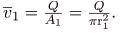Substituting known values and making appropriate unit conversions yieldsSolution for (b)
We could repeat this calculation to find the speed in the nozzle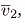, but we will use the equation of continuity to give a somewhat different insight. Using the equation which states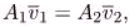solving for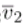and substituting πr2 for the cross-sectional area yields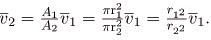Substituting known values,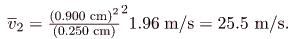Discussion
A speed of 1.96 m/s is about right for water emerging from a nozzleless hose. The nozzle produces a considerably faster stream merely by constricting the flow to a narrower tube.
The solution to the last part of the example shows that speed is inversely proportional to the square of the radius of the tube, making for large effects when radius varies. We can blow out a candle at quite a distance, for example, by pursing our lips, whereas blowing on a candle with our mouth wide open is quite ineffective.
In many situations, including in the cardiovascular system, branching of the flow occurs. The blood is pumped from the heart into arteries that subdivide into smaller arteries (arterioles) which branch into very fine vessels called capillaries. In this situation, continuity of flow is maintained but it is the sum of the flow rates in each of the branches in any portion along the tube that is maintained. The equation of continuity in a more general form becomes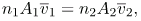where n1 and n2 are the number of branches in each of the sections along the tube.
Calculating Flow Speed and Vessel Diameter: Branching in the Cardiovascular System
The aorta is the principal blood vessel through which blood leaves the heart in order to circulate around the body.
(a) Calculate the average speed of the blood in the aorta if the flow rate is 5.0 L/min. The aorta has a radius of 10 mm.
(b) Blood also flows through smaller blood vessels known as capillaries. When the rate of blood flow in the aorta is 5.0 L/min, the speed of blood in the capillaries is about 0.33 mm/s. Given that the average diameter of a capillary is 8.0μm, calculate the number of capillaries in the blood circulatory system.
Strategy
We can use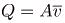to calculate the speed of flow in the aorta and then use the general form of the equation of continuity to calculate the number of capillaries as all of the other variables are known.
Solution for (a)

The flow rate is given by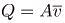or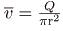for a cylindrical vessel.
Substituting the known values (converted to units of meters and seconds) gives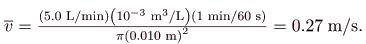Solution for (b)
Using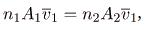assigning the subscript 1 to the aorta and 2 to the capillaries, and solving for n2 (the number of capillaries) gives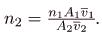Converting all quantities to units of meters and seconds and substituting into the equation above gives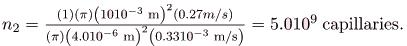Discussion

Note that the speed of flow in the capillaries is considerably reduced relative to the speed in the aorta due to the significant increase in the total cross-sectional area at the capillaries. This low speed is to allow sufficient time for effective exchange to occur although it is equally important for the flow not to become stationary in order to avoid the possibility of clotting. Does this large number of capillaries in the body seem reasonable? In active muscle, one finds about 200 capillaries per mmor about 200106  per 1 kg of muscle. For 20 kg of muscle, this amounts to about 4109 capillaries.
Section Summary

• Flow rate Q is defined to be the volume V flowing past a point in time t, or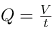where V is volume and t is time.
• The SI unit of volume is m3.
• Another common unit is the liter (L), which is 10-3 m3.
• Flow rate and velocity are related by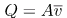where A is the cross-sectional area of the flowand its average velocity.
• For incompressible fluids, flow rate at various points is constant. That is,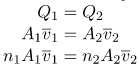Conceptual Questions

1. What is the difference between flow rate and fluid velocity? How are they related?
2. Many figures in the text show streamlines. Explain why fluid velocity is greatest where streamlines are closest together. (Hint: Consider the relationship between fluid velocity and the cross-sectional area through which it flows.)
3. Identify some substances that are incompressible and some that are not.

Problems & Exercises

1. What is the average flow rate in cm3/s of gasoline to the engine of a car traveling at 100 km/h if it averages 10.0 km/L?
2.78 cm3
2. The heart of a resting adult pumps blood at a rate of 5.00 L/min.
(a) Convert this to cm3/s.
(b) What is this rate in m3/s?
3. Blood is pumped from the heart at a rate of 5.0 L/min into the aorta (of radius 1.0 cm). Determine the speed of blood through the aorta.
27 cm/s
4. Blood is flowing through an artery of radius 2 mm at a rate of 40 cm/s. Determine the flow rate and the volume that passes through the artery in a period of 30 s.

The Huka Falls on the Waikato River is one of New Zealand’s most visited natural tourist attractions. On average the river has a flow rate of about 300,000 L/s. At the gorge, the river narrows to 20 m wide and averages 20 m deep.
(a) What is the average speed of the river in the gorge?
(b) What is the average speed of the water in the river downstream of the falls when it widens to 60 m and its depth increases to an average of 40 m?
The Huka Falls in Taupo, New Zealand, demonstrate flow rate. (credit: RaviGogna, Flickr)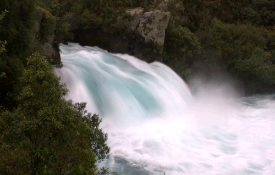(a) 0.75 m/s
(b) 0.13 m/s
A major artery with a cross-sectional area of 1.00 cm2 branches into 18 smaller arteries, each with an average cross-sectional area of 0.400 cm2 . By what factor is the average velocity of the blood reduced when it passes into these branches?
(a) As blood passes through the capillary bed in an organ, the capillaries join to form venules (small veins). If the blood speed increases by a factor of 4.00 and the total cross-sectional area of the venules is 10.0 cm2, what is the total cross-sectional area of the capillaries feeding these venules?
(b) How many capillaries are involved if their average diameter is 10.0 μm?
(a) 40.0 cm2
(b) 5.09107
The human circulation system has approximately 1109 capillary vessels. Each vessel has a diameter of about 8 μm. Assuming cardiac output is 5 L/min, determine the average velocity of blood flow through each capillary vessel.
(a) Estimate the time it would take to fill a private swimming pool with a capacity of 80,000 L using a garden hose delivering 60 L/min.
(b) How long would it take to fill if you could divert a moderate size river, flowing at 5000 m3/s, into it?
(a) 22 h
(b) 0.016 s
The flow rate of blood through a 2.0010-6 -m -radius capillary is 3.80109 m3/s.
(a) What is the speed of the blood flow? (This small speed allows time for diffusion of materials to and from the blood.)
(b) Assuming all the blood in the body passes through capillaries, how many of them must there be to carry a total flow of 90.0 m3/s? (The large number obtained is an overestimate, but it is still reasonable.)
(a) What is the fluid speed in a fire hose with a 9.00-cm diameter carrying 80.0 L of water per second?
(b) What is the flow rate in cubic meters per second
(c) Would your answers be different if salt water replaced the fresh water in the fire hose?
(a) 12.6 m/s
(b) 0.0800 m3/s
(c) No, independent of density.
The main uptake air duct of a forced air gas heater is 0.300 m in diameter. What is the average speed of air in the duct if it carries a volume equal to that of the house’s interior every 15 min? The inside volume of the house is equivalent to a rectangular solid 13.0 m wide by 20.0 m long by 2.75 m high.
Water is moving at a velocity of 2.00 m/s through a hose with an internal diameter of 1.60 cm.
(a) What is the flow rate in liters per second?
(b) The fluid velocity in this hose’s nozzle is 15.0 m/s. What is the nozzle’s inside diameter?
(a) 0.402 L/s
(b) 0.584 cm
Prove that the speed of an incompressible fluid through a constriction, such as in a Venturi tube, increases by a factor equal to the square of the factor by which the diameter decreases. (The converse applies for flow out of a constriction into a larger-diameter region.)
Water emerges straight down from a faucet with a 1.80-cm diameter at a speed of 0.500 m/s. (Because of the construction of the faucet, there is no variation in speed across the stream.)
(a) What is the flow rate in cm3/s?
(b) What is the diameter of the stream 0.200 m below the faucet? Neglect any effects due to surface tension
(a) 127 m3/s
(b) 0.890 cm
Unreasonable Results
A mountain stream is 10.0 m wide and averages 2.00 m in depth. During the spring runoff, the flow in the stream reaches 100,000 m3/s.
(a) What is the average velocity of the stream under these conditions?
(c) What is unreasonable or inconsistent about the premises?

Offer running on EduRev: Apply code STAYHOME200 to get INR 200 off on our premium plan EduRev Infinity!

,

,

,

,

,

,

,

,

,

,

,

,

,

,

,

,

,

,

,

,

,

;